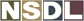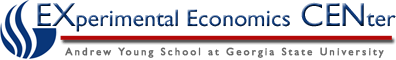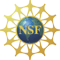Students / Subjects# Lecture to Accompany Tax Experiment

The lecture material is developed on the assumption that students have previously been introduced to step function market supply and demand functions that correspond to goods traded in discrete units (as in everyday life).

Textbook derivations of key results on the theory of taxation are developed using linear supply and demand graphs. Parallel derivations of some of the results are developed using demand and supply step functions.

## Definitions

• The liability to pay a tax falls on the economic agents who are legally liable to pay the tax to the government.

• The incidence of a tax falls on the economic agents whose real incomes are reduced by the tax.

• The deadweight loss or excess burden of a tax is the amount by which the economic agents' loss in real income due to the tax exceeds the tax revenue.

## Central Results in the Theory of Taxes

Given the simplifying assumption of zero transactions costs, one can show theoretically that:

• Except for limiting special cases, a tax has a non-zero deadweight loss.

• The incidence (on buyers and sellers) of a tax is independent of whether the liability to pay the tax is on buyers or sellers.

• The relative incidence (on buyers and sellers) of a tax is determined by relative price elasticities of demand and supply.

## Market Equilibrium with No Tax

Define Q(p) as the market demand function. For example, if the demand function is linear then

Q(p) = a - bp, for a>0 and b>0.

Define P(q) as the inverse market demand function. For example, the inverse of the above linear market demand function is

P(q) = a/b - (1/b)q.

Define Q(p) as the market supply function. For example, if the supply function is linear then

Q(p) =+p, for>0.

Define P(q) as the inverse market supply function. For example, the inverse of the above linear market supply function is

P(q) =/+ (1/)q.

In the absence of taxes and subsidies, the equilibrium (market clearing) condition can be written as

Q(p) = Q(p)

or as

P(q) = P(q).Figure 1. Equilibrium with Linear Demand and Supply and No TaxFigure 2. Equilibrium with Step Function Demand and Supply and No Tax

## Market Equilibrium with a Per Unit Tax

Now assume that a tax of t per unit is imposed on the commodity. In the presence of the tax, the total amount per unit that buyers must pay (the demand price) exceeds the total amount per unit that sellers get to keep (the supply price) by the amount of the tax per unit:

P= P+ t

With tax per unit of t, the market clearing condition can be written as

P(q) = P(q) + t

or as

Q(p) = Q(p),

which can be rewritten as

Q(p) = Q(p+ t)

or as

Q(p- t) = Q(p)Figure 3. Equilibrium with Linear Demand and Supply and a Per Unit TaxFigure 4. Equilibrium with Step Function Demand and Supply and a Per Unit Tax

## Deadweight Loss of a Tax

Except in limiting special cases, a tax imposes a deadweight loss or excess burden on buyers and sellers. The deadweight loss is the amount by which the reduction in buyers' surplus and sellers' surplus exceeds the tax revenue.Figure 5. Deadweight Loss with Linear Demand and Supply and a Per Unit TaxFigure 6. Deadweight Loss with Step Function Demand and Supply and a Per Unit Tax

## Equal Incidence of a Tax with Symmetric Demand and Supply

Regardless of whether the liability to pay a tax falls on buyers or on sellers, the incidence of the tax falls on both sides of the market.

The most direct way to see this central result in tax theory is to observe that the following diagram is the same whether the liability to pay the tax falls on buyers or sellers.

The only thing that is relevant is that the tax drives a wedge between the total amount per unit that buyers must pay (the demand price) and the total amount per unit that sellers get to keep (the supply price) in the amount of the tax per unit:

P= P+ tFigure 7. BL is the Buyers' Loss from the tax and SL is the Sellers' Loss. In this case of symmetric demand and supply, BL = SL regardless of whether the tax liability falls on buyers or sellers.

## Unequal Incidence of a Tax with Asymmetric Demand and Supply

We have observed that the incidence of a tax is independent of whether the liability to pay the tax is on buyers or sellers.

If tax liability doesn't determine tax incidence then what does? Relative price elasticities of demand and supply determine tax incidence, as we shall now explain.Figure 8. If demand is less elastic than supply then tax incidence falls more on buyers than on sellers.Figure 9. If supply is less elastic than demand then tax incidence falls more on sellers than on buyers.Figure 10. If supply is perfectly inelastic then tax incidence falls entirely on sellers.Figure 11. If supply is perfectly elastic then tax incidence falls entirely on buyers.Figure 12. If demand is perfectly inelastic then tax incidence falls entirely on buyers.